Factor Tree

Chapter 3 Class 6 Playing with Numbers
Concept wise

Another method to write factors are factor tree

Let us find factor tree of 24

24 = 2 × 12

= 2 × 2 × 6

= 2 × 2 × 2 × 3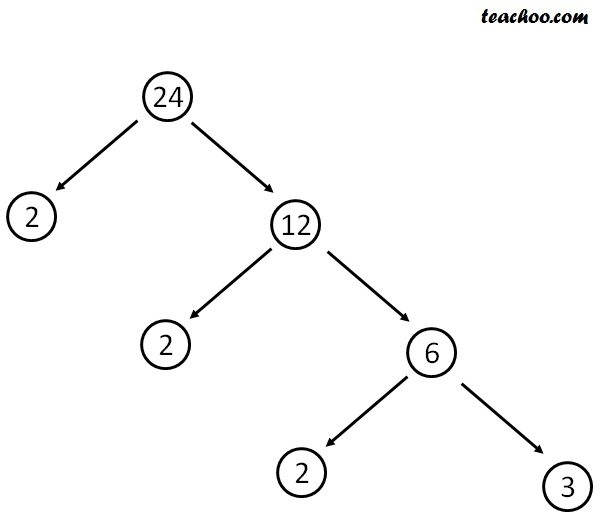Another can be

24 = 3 × 8

= 3 × 2 × 4

= 3 × 2 × 2 × 2Another can be

24 = 4 × 6

= 2 × 2 × 6

= 2 × 2 × 2 × 3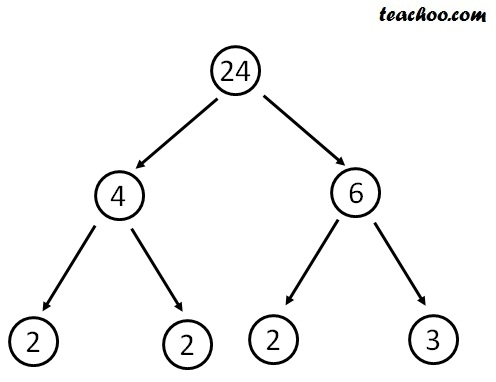## Factor Tree of 36

36 = 2 × 18

= 2 × 9 × 2

= 2 × 3 × 3 × 2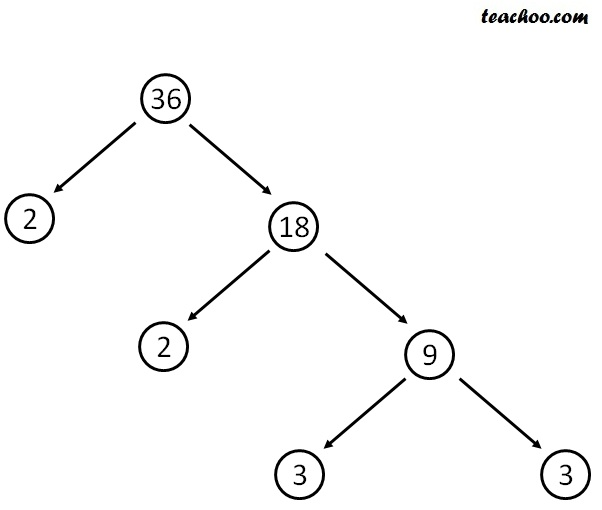Another can be

36 = 3 × 12

= 3 × 6 × 2

= 3 × 2 × 3 × 2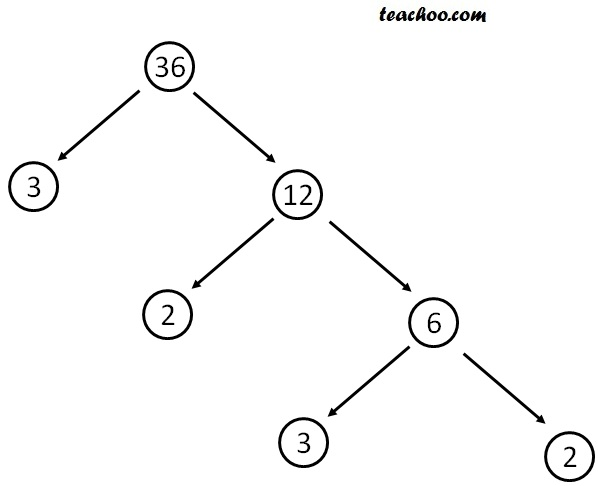Another can be

36 = 6 × 6

= 2 × 3 × 6

= 2 × 3 × 2 × 3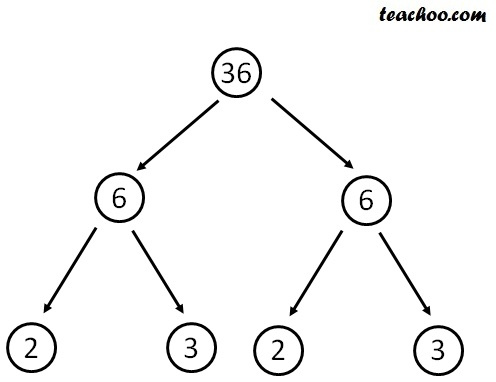Another can be

36 = 4 × 9

= 2 × 2 × 9

= 2 × 2 × 3 × 3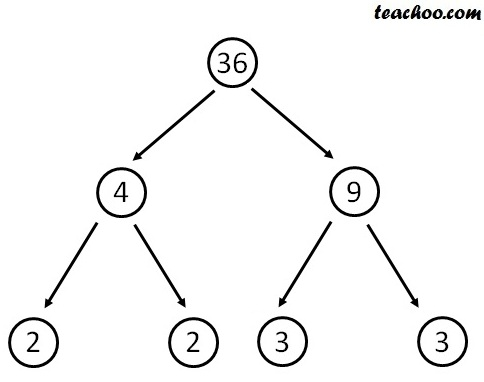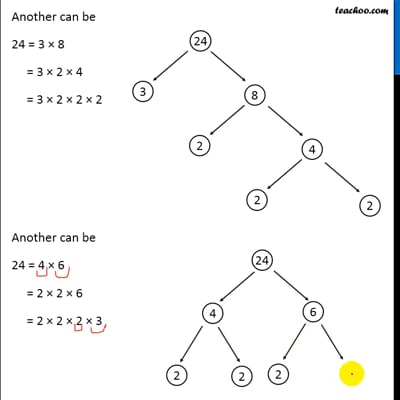This video is only available for Teachoo black users

Introducing your new favourite teacher - Teachoo Black, at only ₹83 per month